Next: Vortex Lines, Vortex Tubes, Up: Incompressible Inviscid Flow Previous: Tidal Bores

# Flow over a Broad-Crested Weir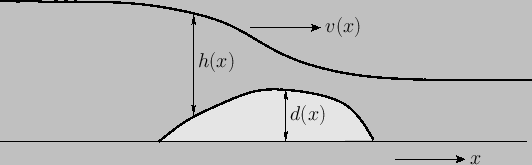Consider the situation, illustrated in Figure 4.8, in which a broad-crested weir is placed in a shallow stream. The purpose of the weir is to impede the flow in such a manner that there is a transition from sub-critical flow, upstream of the weir, to super-critical flow, immediately downstream of the weir. (There is usually a transition back to sub-critical flow, via a hydraulic jump, some way downstream of the weir.)

Letmeasure horizontal distance, and let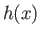,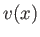, and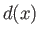be the stream depth, stream velocity, and the height of the weir above the stream bed, respectively. (Here, we are assuming that the velocity remains uniform across the stream at any point on the weir.) A direct generalization of the analysis of Section 4.7 reveals that(4.64)

and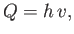(4.65)

where (via continuity) the flow rate,, is not a function of. It follows that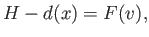(4.66)

where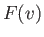is defined in Equation (4.21). Suppose that the weir attains its maximum height,, at. It follows that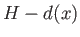passes through a minimum value at. Hence, we would expectto also pass through its minimum value at. It follows that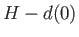(4.67)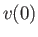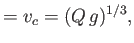(4.68)

where use has been made of Equations (4.22) and (4.23). However,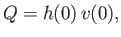(4.69)

where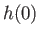is the depth of the stream as its passes over the highest point of the weir. Thus, we deduce that(4.70)

In other words, the flow rate (per unit width) is very simply related to the depth of the stream as it passes over the highest point of the weir, or, alternatively, the depth of the highest point of the weir below the water surface in the reservoir feeding the stream. For this reason, weirs are commonly used as devices to both measure and control flow rates in streams.Next: Vortex Lines, Vortex Tubes, Up: Incompressible Inviscid Flow Previous: Tidal Bores
Richard Fitzpatrick 2016-03-31